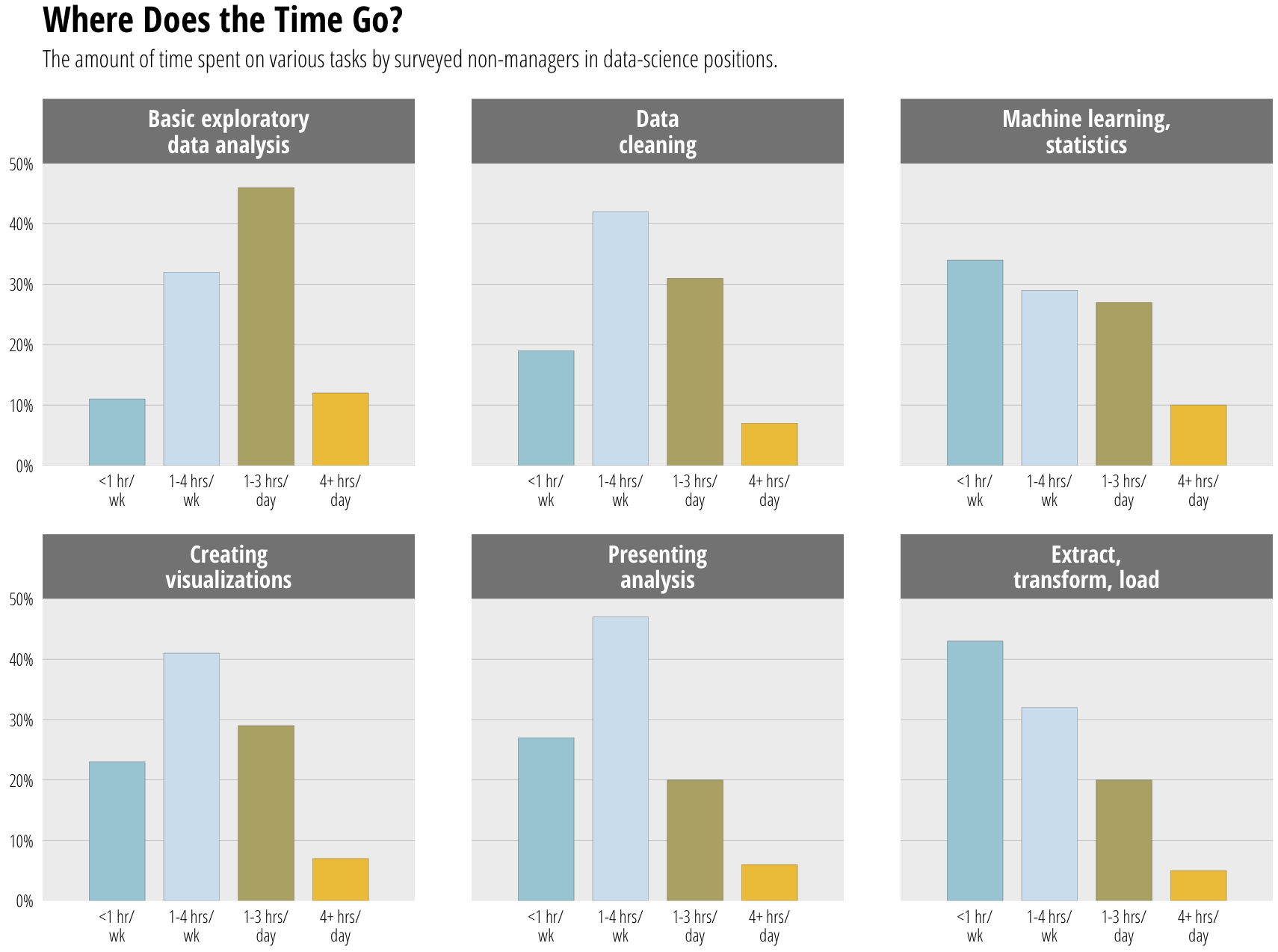# Spinning Cycles in Box #4 To Take the Pies out of Pi Day

>UPDATE: time spent per task factor order was wrong before. now fixed.

I caught this tweet today:

The WSJ folks usually do a great job, but this was either rushed or not completely thought through. There’s no way you’re going to be able to do any real comparisons between the segments across pies and direct pie % labels kinda mean they should have just made a table if they were going to phone it in.

Despite the fact that today is Pi[e] Day, these pies need to go.

If the intent was to primarily allow comparison of hours in-task, leaving some ability to compare the same time category across tasks, then bars are probably the way to go (you could do a parallel coordinates plot, but those looks like tangled guitar strings to me, so I’ll stick with bars). Here’s one possible alternative using R & ggplot2. Since I provide the data, please link to your own creations as I’d love to see how others would represent the data.

NOTE: I left direct bar labels off deliberately. My view is that (a) this is designed to be a relative comparison vs precise comparison & (b) it’s survey data and if we’re going to add #’s I’d feel compelled to communicate margin of error, etc. I don’t think that’s necessary.

```library(ggplot2)
library(grid)
library(scales)
library(hrbrmisc) # devtools::install_github("hrbrmstr/hrbrmisc")
library(tidyr)

Basic exploratory data analysis|11|32|46|12
Data cleaning|19|42|31|7
Machine learning, statistics|34|29|27|10
Creating visualizations|23|41|29|7
Presenting analysis|27|47|20|6

amount_trans <- c("less_than_one_hour_per_week"="<1 hr/\nwk",
"one_to_four_hours_per_week"="1-4 hrs/\nwk",
"one_to_three_hours_a_day"="1-3 hrs/\nday",
"four_or_more_hours_a_day"="4+ hrs/\nday")

dat <- gather(dat, amount, value, -Task)
dat\$value <- dat\$value / 100
dat\$amount <- factor(amount_trans[dat\$amount], levels=amount_trans)

title_trans <- c("Basic exploratory data analysis"="Basic exploratory\ndata analysis",
"Data cleaning"="Data\ncleaning",
"Machine learning, statistics"="Machine learning,\nstatistics",
"Creating visualizations"="Creating\nvisualizations",
"Presenting analysis"="Presenting\nanalysis",

gg <- ggplot(dat, aes(x=amount, y=value, fill=amount))
gg <- gg + geom_bar(stat="identity", width=0.75, color="#2b2b2b", size=0.05)
gg <- gg + scale_y_continuous(expand=c(0,0), labels=percent, limits=c(0, 0.5))
gg <- gg + scale_x_discrete(expand=c(0,1))
gg <- gg + scale_fill_manual(name="", values=c("#a6cdd9", "#d2e4ee", "#b7b079", "#efc750"))
gg <- gg + facet_wrap(~Task, scales="free")
gg <- gg + labs(x=NULL, y=NULL, title="Where Does the Time Go?")
gg <- gg + theme_hrbrmstr(grid="Y", axis="x", plot_title_margin=9)
gg <- gg + theme(panel.background=element_rect(fill="#efefef", color=NA))
gg <- gg + theme(strip.background=element_rect(fill="#858585", color=NA))
gg <- gg + theme(strip.text=element_text(family="OpenSans-CondensedBold", size=12, color="white", hjust=0.5))
gg <- gg + theme(panel.margin.x=unit(1, "cm"))
gg <- gg + theme(panel.margin.y=unit(0.5, "cm"))
gg <- gg + theme(legend.position="none")
gg <- gg + theme(panel.grid.major.y=element_line(color="#b2b2b2"))
gg <- gg + theme(axis.text.x=element_text(margin=margin(t=-10)))
gg <- gg + theme(axis.text.y=element_text(margin=margin(r=-10)))

ggplot_with_subtitle(gg,
"The amount of time spent on various tasks by surveyed non-managers in data-science positions.",
fontfamily="OpenSans-CondensedLight", fontsize=12, bottom_margin=16)```Amazon Author Page

## 4 Comments →Spinning Cycles in Box #4 To Take the Pies out of Pi Day

1.Barry

Thanks for posting this! I’m keeping it as an example.

(BTW, these guys seem to spend less time on grunt work than I would have expected)

2.Carl Witthoft

Yeah, Wapo, even before being bought out and neutered by a certain newsmedia company, insisted on posting moronic pie charts like these.

3.patternprojectyahoocom

Nice theme, I am going to steal it …

This site uses Akismet to reduce spam. Learn how your comment data is processed.